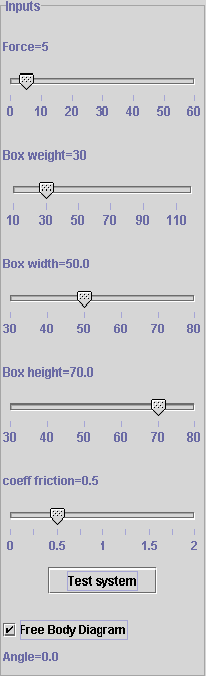search UMD   This siteIf the applet doesnt load, you may need to install the appropriate plugins, a link to the plugins can be found
 User’s Guide The model represents the sliding-Tipping mechanism where the motion of the weight box is determined by the force applied to the box, weight of the box, dimensions of the box, reaction to the weight and coefficient of the friction. The simulation model uses the equilibrium equations as follows: SFx=FA-Wx-Fr=0 SFy= Wy-N=0 SM=Wx*x + Wy*h/2-FA*h=0 FA=Applied force                                                       Fr=Frictional force Wx=Weight component along x-axis                        Wy=Weight component along y-axis  h=Height of the block                                                 N=Reaction to the weight of the block x= location along the x-axis where the “Reaction” (N) is acting. The maximum frictional force is Fr=m*N where m is coefficient of friction.Using the different sliders shown at the right side of applet screen, the following parameters can be changed: Applied force Box weight Box width Box height Coefficient of friction On checking the checkbox in front of Free Body Diagram the free body diagram can be viewed for that particular case depending on the values of the parameters described above. The Test button on clicking starts the process of testing the system and shows the “reset” text on button that can be clicked to reset initial values.